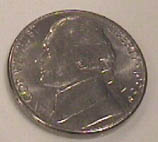# A Nickel's Worth of RadiationAs an example of the kind of things you can model with the Planck radiation formula, consider the following questions: How much radiant energy comes from a nickel at room temperature per second? How many photons per second leave the nickel? What volume of air would it take to have energy equal to 1 second of radiation from the nickel? What volume of the room would have radiation energy equal to 1 second of radiation from the nickel?

Measured properties of the nickel are diameter = 2.14 cm, thickness 0.2 cm, mass 5.1 grams. This gives a volume of 0.719 cm3 and a surface area of 8.54 cm 2. This gives a calculated density of 7.09 gm/cm3 compared to the density of 8.9 gm/cm3 for the pure element from the periodic table. So either the material is not pure nickel, being alloyed with some lighter element, or the measurement of thickness is off, which is a real possibility.

1. How much radiant energy comes from a nickel at room temperature per second?

The radiation from the nickel's surface can be calculated from the Stefan Boltzmann law. The room temperature will be taken to be 22°C = 295 K. Assuming an ideal radiator for this estimate, the radiated power is

P = σAT4 = (5.67 x 10-8 W/m2K4)x(8.54 x 10-4 m2)x(295 K)4 = 0.367 watts.

So the radiated power from a nickel at room temperature is about 0.37 watts.

2. How many photons per second leave the nickel?

Since we know the energy, we can divide it by the average photon energy. We don't know a true average, but the wavelength of the peak of the blackbody radiation curve is a representative value which can be used as an estimate. This may be obtained from the Wien displacement law.

λpeak = 0.0029 m K/295 K = 9.83 x 10-6 m = 9830 nm, in the infrared.

The energy per photon at this peak can be obtained from the Planck relationship.

Ephoton = hυ = hc/λ = 1240 eV nm/ 9830 nm = 0.126 eV

Then the number of photons per second is

N = (0.367 J)/(0.126 eV x 1.6 x 10-19 J/eV) = 1.82 x 1019 photons

3. What volume of air would it take to have energy equal to 1 second of radiation from the nickel?

The translational kinetic energy of an air molecule can be obtained from equipartition of energy and the definition of the kinetic temperature.

Kinetic energyaverage = 3/2 kT = 3/2 (8.617 x 10-5 eV/K)(295 K) = 0.038 eV

The number of molecules to equal one second's worth of radiation is then

Nmolecules = (0.367 J)/(0.038 eV x 1.6 x 10-19 J/eV) = 6.0 x 1019 molecules

The volume per air molecule can be found by using the fact that a mole of an ideal gas occupies 22.4 liters at STP. Using the ideal gas law

Vair molecule = (22.4 x 10-3 m3)(295K/273K)/(6.02 x 1023 molecules/mole) = 4.02 x 10-26m3

Volume of air to have 0.367 J =(4.02 x 10-26m3)(6.0 x 1019 molecules) = 2.41x 10-6m3

So the small volume of about 2.4 cm3 of air will have kinetic energy equal to the amount of energy radiated from the nickel per second.

4. What volume of the room would have radiation energy equal to 1 second of radiation from the nickel?

The Stefan-Boltzmann relationship is also related to the energy density in the radiation in a given volume of space. The energy density is

4σAT4/c = 4(5.67 x 10-8 W/m2K4)x(295 K)4/(3 x 108m/s)

Energy density in radiation = 5.73 x 10-6J/m3

So the volume required is (0.367 J)/(5.73 x 10-6J/m3) = 64000 m3. This is the volume of a cube with dimension 40 meters per side.

The air molecules in a volume of air about three times the volume of the nickel will have kinetic energy equal to the radiation from the nickel, but it takes the radiation content of a large auditorium to equal that amount. The ratio of the energy per unit volume in the molecular kinetic energy is more than 1010 times that in radiation.

 Applications of the Planck radiation formula What about the division of energy between mass and radiation in the universe?
Index